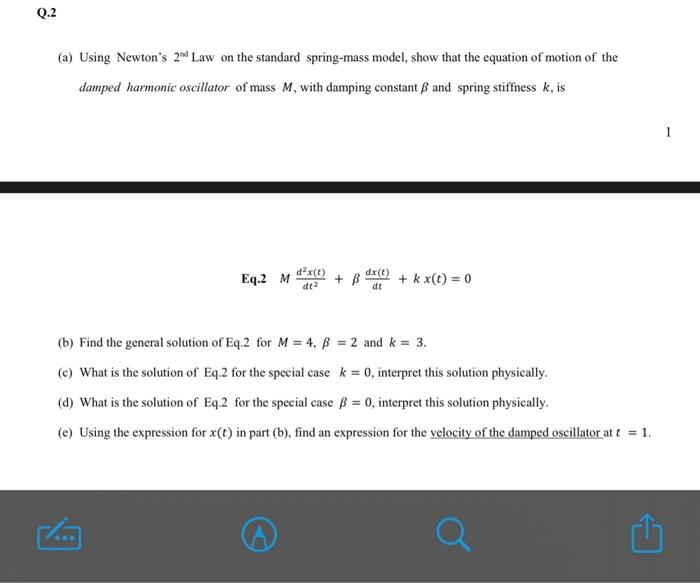Home / Expert Answers / Mechanical Engineering / q-2-a-using-newton-39-s-2nd-law-on-the-standard-spring-mass-model-show-that-the-equation-of-motion-o-pa540

# (Solved): Q.2 (a) Using Newton's 2nd Law on the standard spring-mass model, show that the equation of motion o ...

Q.2 (a) Using Newton's 2nd Law on the standard spring-mass model, show that the equation of motion of the damped harmonic oscillator of mass M, with damping constant B and spring stiffness k, is Eq.2 M 0.0 d²x(t) dt² + B dx (t) dt + kx(t) = 0 (b) Find the general solution of Eq.2 for M = 4, B = 2 and k = 3. (c) What is the solution of Eq.2 for the special case k = 0, interpret this solution physically. (d) What is the solution of Eq.2 for the special case ß = 0, interpret this solution physically. (e) Using the expression for x(t) in part (b), find an expression for the velocity of the damped oscillator at t = 1. 1 M(a) Using Newton's Law on the standard spring-mass model, show that the equation of motion of the damped harmonic oscillator of mass , with damping constant and spring stiffness , is Eq.2 (b) Find the general solution of Eq.2 for and . (c) What is the solution of Eq.2 for the special case , interpret this solution physically. (d) What is the solution of Eq.2 for the special case , interpret this solution physically. (e) Using the expression for in part (b), find an expression for the velocity of the damped oscillator at .

We have an Answer from Expert